# Introduction to Machine Learning

Machine Learning is the most need-based technology in today’s world. It’s application range is so broad that is used in self-driving cars and also to detect many severe diseases. So, we can say that Machine Learning deals with both technical and medical fields, in this way Machine Learning makes a correlation among different scientific domains.

The term Machine Learning was coined by Arthur Samuel in 1959,an American pioneer in the field of computer gaming, artificial intelligence and stated that “It gives computers the ability to learn without being explicitly programmed”.

In 1997, Tom Mitchel gave a well-posed mathematical and relational definition that “A computer program is said to learn from experience E with respect to some task T and some performance measure P, if its performance on T, as measured by P, improves with experience E.”

In simple way, I can say that Machine Learning is a process by which Machine is automatically trained from different kinds of datasets and we check it’s accuracy level by it’s performance. In the sense, it is the practice of getting Machines to solve problems by gaining the ability to think. By continuous process of thinking the machine improves it’s accuracy level.Machine learning techniques leverage data mining to identify historic trends to inform future models.

i)Machine Learning Algorithm- A Machine Learning algorithm is a set of rules and techniques used to learn patterns from data and draw significant information from it. It is the main logic behind Machine Learning. An example of a Machine Learning algorithm is the Linear Regression Algorithm.The typical supervised machine learning algorithm consists of (roughly) three components:

1. A decision process: A recipe of calculations or other steps that takes in the data and returns a “guess” at the kind of pattern in the data your algorithm is looking to find.
2. An error function: A method of measuring how good the guess was by comparing it to known examples (when they are available). Did the decision process get it right? If not, how do you quantify “how bad” the miss was?
3. An optimization process: Where the algorithm looks at the miss and then updates how the decision process comes to the final decision so that the next time the miss won’t be as great.

ii)Model- A model is the main component of Machine Learning. A model is trained by using Machine Learning Algorithm. An algorithm maps all the decisions that a model is supposed to take based on the given input, in order to get the correct output.

iii)Predictor Variable- It is used to predict the output.

iv)Response Variable- It is the feature of the output variable that needs to be predicted by using the Predictor Variable.

v)Training Data- Using Training Data, Machine Learning Model is built and this data helps the model to identify key trends , patterns and styles required to predict the output.

vi)Testing Data- After the model is trained, it must be tested to evaluate how accurately it can predict an outcome.

vi)Accuracy- It is one metric which is used to evaluate classification models. Accuracy is the fraction of predictions our model got right. Accuracy is (Number of correct predictions)/(Total number of predictions). For binary classification , accuracy can also be calculated in terms of positive and negative as follows:

Accuracy=( TP + TN)/(TP+TN+FP+FN)

Where TP=True Positives, TN=True Negatives, FP=False Positives, FN=False Negatives.

• Increase in Data Generation: Due to excessive production of data, we need a method that can be used to structure, analyze and draw useful insights from data. This is where Machine Learning comes in. It uses data to solve problems and find solutions to the most complex tasks faced by organizations.
• Improve Decision Making: By making use of various algorithms, Machine Learning can be used to make better business decisions. For example, Machine Learning is used to forecast sales, predict downfalls in the stock market, identify risks and anomalies, etc.
• Uncover patterns & trends in data: Finding hidden patterns and extracting key insights from data is the most essential part of Machine Learning. By building predictive models and using statistical techniques, Machine Learning allows you to dig beneath the surface and explore the data at a minute scale. Understanding data and extracting patterns manually will take days, whereas Machine Learning algorithms can perform such computations in less than a second.
• Solve complex problems: From detecting the genes linked to the deadly ALS disease to building self-driving cars, Machine Learning can be used to solve the most complex problems.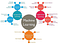Machine Learning Classification

Machine learning implementations are classified into three major categories, depending on the nature of the learning “signal” or “response” available to a learning system which are as follows:-

“Supervised learning is a technique in which we teach or train the machine using data which is well labeled.”

The data set being used has been pre-labeled and classified by users to allow the algorithm to see how accurate its performance is.This approach is indeed similar to human learning under the supervision of a teacher. The teacher provides good examples for the student to memorize, and the student then derives general rules from these specific examples.

1. Classification- When inputs are divided into two or more classes, and the learner must produce a model that assigns unseen inputs to one or more (multi-label classification) of these classes. This is typically tackled in a supervised way. Spam filtering is an example of classification, where the inputs are email (or other) messages and the classes are “spam” and “not spam”.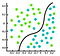Classification

a)Strengths: Classification tree perform very well in practice

b)Weaknesses: Unconstrained, individual trees are prone to over-fitting.

2.Regression-Regression technique predicts a single output value using training data.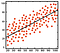Regression

a)Strengths: Outputs always have a probabilistic interpretation, and the algorithm can be regularized to avoid over-fitting.

b)Weaknesses: Logistic regression may under-perform when there are multiple or non-linear decision boundaries. This method is not flexible, so it does not capture more complex relationships.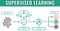Supervised Machine Learning

“Unsupervised learning involves training by using unlabeled data and allowing the model to act on that information without guidance.”

The raw data set being used is unlabeled and an algorithm identifies patterns and relationships within the data without help from users.As a kind of learning, it resembles the methods humans use to figure out that certain objects or events are from the same class, such as by observing the degree of similarity between objects. Some recommendation systems that you find on the web in the form of marketing automation are based on this type of learning.

1. Clustering- It is the assignment of a set of observations into subsets (called clusters) so that observations in the same cluster are similar in some sense. Clustering is a method of unsupervised learning, and a common technique for statistical data analysis used in many fields.
2. Dimensionality reduction- It refers to techniques that reduce the number of input variables in a data set. … Large numbers of input features can cause poor performance for machine learning algorithms. Dimensionality reduction is a general field of study concerned with reducing the number of input features.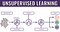Unsupervised Machine Learning

The data set contains structured and unstructured data, which guide the algorithm on its way to making independent conclusions. The combination of the two data types in one training data set allows machine learning algorithms to learn to label unlabeled data.There is a special case of this principle known as Transduction where the entire set of problem instances is known at learning time, except that part of the targets are missing.

“Reinforcement Learning is a part of Machine learning where an agent is put in an environment and he learns to behave in this environment by performing certain actions and observing the rewards which it gets from those actions.”

The data set uses a "rewards/punishments" system, offering feedback to the algorithm to learn from its own experiences by trial and error.In this case, an application presents the algorithm with examples of specific situations, such as having the gamer stuck in a maze while avoiding an enemy. The application lets the algorithm know the outcome of actions it takes, and learning occurs while trying to avoid what it discovers to be dangerous and to pursue survival. You can have a look at how the company Google Deep Mind has created a reinforcement learning program that plays old Atari’s video games. When watching the video, notice how the program is initially clumsy and unskilled but steadily improves with training until it becomes a champion.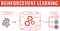Reinforcement Machine Learning

The Machine Learning process involves building a Predictive model that can be used to find a solution for a Problem Statement. To understand the Machine Learning process let’s assume that you have been given a problem that needs to be solved by using Machine Learning.

The below steps are followed in a Machine Learning process:

Step 1: Define the objective of the Problem Statement- Through this step we able to understand what we have to predict. Understanding the target prediction we can make a strategy by which we able to solve the problem.

Step 2: Data Gathering- In this step we have to collect appropriate and perfect data by which we can solve the problem.

Step 3:Data Preparation- The data we collected may be not in right format.We will encounter a lot of inconsistencies in the data set such as missing values, redundant variables, duplicate values(dummies), etc. Removing such inconsistencies is very essential because they might lead to wrongful computations and predictions. So, In this step we have to filter out the imperfects and prepare perfect,error less data.

Step 4: Exploratory Data Analysis(EDA)-This is a brainstorming stage of Machine Learning. In this stage we have to dive deep into data and find all the hidden data mysteries. Data Exploration involves understanding the patterns and trends in the data. At this stage, all the useful insights are drawn and correlations between the variables are understood.

Step 5: Building a Machine Learning Model- This stage always begins by splitting the data set into two parts, training data, and testing data. The training data will be used to build and analyze the model. The logic of the model is based on the Machine Learning Algorithm that is being implemented.

Step 6: Model Evaluation & Optimization- After building a model by using the training data set, it is finally time to put the model to a test. The testing data set is used to check the efficiency of the model and how accurately it can predict the outcome. Once the accuracy is calculated, any further improvements in the model can be implemented at this stage. Methods like parameter tuning and cross-validation can be used to improve the performance of the model.

Step 7: Predictions- Once the model is evaluated and improved, it is finally used to make predictions. The final output can be a Categorical variable (eg. True or False) or it can be a Continuous Quantity (eg. the predicted value of a stock).

• Netflix’s Recommendation Engine: The core of Netflix is its infamous recommendation engine. Over 75% of what you watch is recommended by Netflix and these recommendations are made by implementing Machine Learning.
• Facebook’s Auto-tagging feature: The logic behind Facebook’s Deep Mind face verification system is Machine Learning and Neural Networks. Deep Mind studies the facial features in an image to tag your friends and family.
• Amazon’s Alexa: The infamous Alexa, which is based on Natural Language Processing and Machine Learning is an advanced level Virtual Assistant that does more than just play songs on your playlist. It can book you an Uber, connect with the other IoT devices at home, track your health, etc.
• Google’s Spam Filter: Gmail makes use of Machine Learning to filter out spam messages. It uses Machine Learning algorithms and Natural Language Processing to analyze emails in real-time and classify them as either spam or non-spam.

Enthusiast in Machine Learning || Deep Learning || B.Tech In CSE

Enthusiast in Machine Learning || Deep Learning || B.Tech In CSE